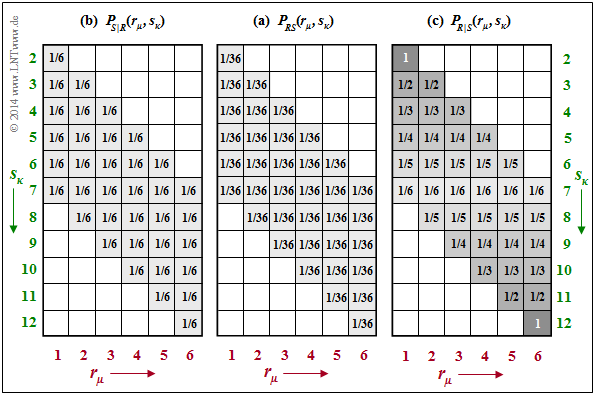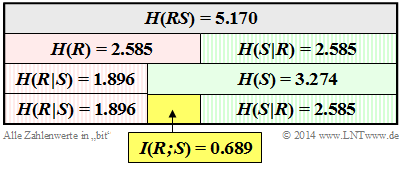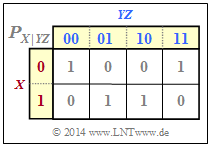# Different Entropy Measures of Two-Dimensional Random Variables

## Definition of entropy using supp(PXY)

We briefly summarise the results of the last chapter again, assuming the two-dimensional random variable  $XY$  with the probability function  $P_{XY}(X,\ Y)$ .  At the same time we use the notation

$${\rm supp} (P_{XY}) = \big \{ \hspace{0.05cm}(x,\ y) \in XY \hspace{0.05cm}, \hspace{0.3cm} {\rm where} \hspace{0.15cm} P_{XY}(X,\ Y) \ne 0 \hspace{0.05cm} \big \} \hspace{0.05cm};$$

$\text{Summarising the last chapter:}$

With this subset $\text{supp}(P_{XY}) ⊂ P_{XY}$ , the following holds for

• the  joint entropy :
$$H(XY) = {\rm E}\hspace{-0.1cm} \left [ {\rm log}_2 \hspace{0.1cm} \frac{1}{P_{XY}(X, Y)}\right ] =\hspace{-0.2cm} \sum_{(x, y) \hspace{0.1cm}\in \hspace{0.1cm}{\rm supp} (P_{XY}\hspace{-0.05cm})} \hspace{-0.6cm} P_{XY}(x, y) \cdot {\rm log}_2 \hspace{0.1cm} \frac{1}{P_{XY}(x, y)} \hspace{0.05cm}.$$
• the  entropies of the 1D random variables  $X$  and  $Y$:
$$H(X) = {\rm E}\hspace{-0.1cm} \left [ {\rm log}_2 \hspace{0.1cm} \frac{1}{P_{X}(X)}\right ] =\hspace{-0.2cm} \sum_{x \hspace{0.1cm}\in \hspace{0.1cm}{\rm supp} (P_{X})} \hspace{-0.2cm} P_{X}(x) \cdot {\rm log}_2 \hspace{0.1cm} \frac{1}{P_{X}(x)} \hspace{0.05cm},$$
$$H(Y) = {\rm E}\hspace{-0.1cm} \left [ {\rm log}_2 \hspace{0.1cm} \frac{1}{P_{Y}(Y)}\right ] =\hspace{-0.2cm} \sum_{y \hspace{0.1cm}\in \hspace{0.1cm}{\rm supp} (P_{Y})} \hspace{-0.2cm} P_{Y}(y) \cdot {\rm log}_2 \hspace{0.1cm} \frac{1}{P_{Y}(y)} \hspace{0.05cm}.$$

$\text{Example 1:}$  We refer again to the examples on the  joint probability and joint entropy  in the last chapter. For the 2D probability function  $P_{RB}(R, B)$  in  $\text{example 5}$  there with the parameters

• $R$   ⇒   numbers of the red die and
• $B$   ⇒   number of the blue die

the sets  $P_{RB}$  and  $\text{supp}(P_{RB})$  are identical.  Here, all  $6^2 = 36$  squares are occupied by non-zero values.

For the 2D probability function  $P_{RS}(R, S)$  in  $\text{example 6}$  mit den Parametern

• $R$   ⇒   numbers of the red die
• $S = R + B$   ⇒   sum of both dice

there are  $6 · 11 = 66$ squares, many of which, however, are empty, i.e. stand for the probability "0" .

• The subset  $\text{supp}(P_{RS})$ , on the other hand, contains only the  $36$  shaded squares with non-zero probabilities.
• The entropy remains the same no matter whether one averages over all elements of  $P_{RS}$  or only over the elements of   $\text{supp}(P_{RS})$ since for  $x → 0$  the limit is  $x · \log_2 ({1}/{x}) = 0$  ist.

## Conditional probability and conditional entropy

In the book "Theory of Stochastic Signals" the following   conditional probabilities  were given for the case of two events  $X$  and  $Y$  ⇒   Bayes' theorem:

$${\rm Pr} (X \hspace{-0.05cm}\mid \hspace{-0.05cm} Y) = \frac{{\rm Pr} (X \cap Y)}{{\rm Pr} (Y)} \hspace{0.05cm}, \hspace{0.5cm} {\rm Pr} (Y \hspace{-0.05cm}\mid \hspace{-0.05cm} X) = \frac{{\rm Pr} (X \cap Y)}{{\rm Pr} (X)} \hspace{0.05cm}.$$

Applied to probability functions, one thus obtains:

$$P_{\hspace{0.03cm}X \mid \hspace{0.03cm} Y} (X \hspace{-0.05cm}\mid \hspace{-0.05cm} Y) = \frac{P_{XY}(X, Y)}{P_{Y}(Y)} \hspace{0.05cm}, \hspace{0.5cm} P_{\hspace{0.03cm}Y \mid \hspace{0.03cm} X} (Y \hspace{-0.05cm}\mid \hspace{-0.05cm} X) = \frac{P_{XY}(X, Y)}{P_{X}(X)} \hspace{0.05cm}.$$

Analogous to the  joint entropy  $H(XY)$ , the following entropy functions can be derived here:

$\text{Definitions:}$

• The  conditional entropy of the random variable  $X$  under condition  $Y$  is:
$$H(X \hspace{-0.05cm}\mid \hspace{-0.05cm} Y) = {\rm E} \hspace{-0.1cm}\left [ {\rm log}_2 \hspace{0.1cm}\frac{1}{P_{\hspace{0.03cm}X \mid \hspace{0.03cm} Y} (X \hspace{-0.05cm}\mid \hspace{-0.05cm} Y)}\right ] = \hspace{-0.2cm} \sum_{(x, y) \hspace{0.1cm}\in \hspace{0.1cm}{\rm supp} (P_{XY}\hspace{-0.08cm})} \hspace{-0.6cm} P_{XY}(x, y) \cdot {\rm log}_2 \hspace{0.1cm} \frac{1}{P_{\hspace{0.03cm}X \mid \hspace{0.03cm} Y} (x \hspace{-0.05cm}\mid \hspace{-0.05cm} y)}=\hspace{-0.2cm} \sum_{(x, y) \hspace{0.1cm}\in \hspace{0.1cm}{\rm supp} (P_{XY}\hspace{-0.08cm})} \hspace{-0.6cm} P_{XY}(x, y) \cdot {\rm log}_2 \hspace{0.1cm} \frac{P_{Y}(y)}{P_{XY}(x, y)} \hspace{0.05cm}.$$
• Similarly, for the  second conditional entropy we obtain:
$$H(Y \hspace{-0.1cm}\mid \hspace{-0.05cm} X) = {\rm E} \hspace{-0.1cm}\left [ {\rm log}_2 \hspace{0.1cm}\frac{1}{P_{\hspace{0.03cm}Y\hspace{0.03cm} \mid \hspace{0.01cm} X} (Y \hspace{-0.08cm}\mid \hspace{-0.05cm}X)}\right ] =\hspace{-0.2cm} \sum_{(x, y) \hspace{0.1cm}\in \hspace{0.1cm}{\rm supp} (P_{XY}\hspace{-0.08cm})} \hspace{-0.6cm} P_{XY}(x, y) \cdot {\rm log}_2 \hspace{0.1cm} \frac{1}{P_{\hspace{0.03cm}Y\hspace{-0.03cm} \mid \hspace{-0.01cm} X} (y \hspace{-0.05cm}\mid \hspace{-0.05cm} x)}=\hspace{-0.2cm} \sum_{(x, y) \hspace{0.1cm}\in \hspace{0.1cm}{\rm supp} (P_{XY}\hspace{-0.08cm})} \hspace{-0.6cm} P_{XY}(x, y) \cdot {\rm log}_2 \hspace{0.1cm} \frac{P_{X}(x)}{P_{XY}(x, y)} \hspace{0.05cm}.$$

In the argument of the logarithm function there is always a conditional probability function   ⇒   $P_{X\hspace{0.03cm}| \hspace{0.03cm}Y}(·)$  bzw.  $P_{Y\hspace{0.03cm}|\hspace{0.03cm}X}(·)$, while the joint probability   ⇒   $P_{XY}(·)$ is needed for the expectation value formation.

For the conditional entropies, there are the following limitations:

• Both  $H(X|Y)$  and  $H(Y|X)$  are always greater than or equal to zero.  From  $H(X|Y) = 0$  it follows directly also  $H(Y|X) = 0$.  Both are only possible for   disjoint sets  $X$  and  $Y$  .
•   $H(X|Y) ≤ H(X)$  and  $H(Y|X) ≤ H(Y)$ always apply.  These statements are plausible if one realises that one can also use "uncertainty" synonymously for "entropy" .  For:   the uncertainty with respect to the quantity  $X$  cannot be increased by knowing  $Y$  .
• Except in the case of statistical independence   ⇒   $H(X|Y) = H(X)$ ,   $H(X|Y) < H(X)$ always holds.  Because of  $H(X) ≤ H(XY)$  and  $H(Y) ≤ H(XY)$ ,   $H(X|Y) ≤ H(XY)$  and  $H(Y|X) ≤ H(XY)$ therefore also hold.   Thus, a conditional entropy can never become larger than the joint entropy.

$\text{Example 2:}$  We consider the joint probabilities  $P_{RS}(·)$  of our dice experiment, which were determined in the  last chapter  als  $\text{example 6}$  .    $P_{RS}(·)$  is given again in the middle of the following graph.Joint probabilities  $P_{RS}$  and conditional probabilities  $P_{S \vert R}$  and  $P_{R \vert S}$

The two conditional probability functions are drawn on the outside:

• Given on the left is the conditional probability function  $P_{S \vert R}(⋅) = P_{SR}(⋅)/P_R(⋅)$.  Because of  $P_R(R) = \big [1/6, \ 1/6, \ 1/6, \ 1/6, \ 1/6, \ 1/6 \big ]$  the same probability value   $1/6$ is here in all shaded fields   ⇒   $\text{supp}(P_{S\vert R}) = \text{supp}(P_{R\vert S})$  .  From this follows for the conditional entropy:
$$H(S \hspace{-0.1cm}\mid \hspace{-0.13cm} R) = \hspace{-0.2cm} \sum_{(r, s) \hspace{0.1cm}\in \hspace{0.1cm}{\rm supp} (P_{RS})} \hspace{-0.6cm} P_{RS}(r, s) \cdot {\rm log}_2 \hspace{0.1cm} \frac{1}{P_{\hspace{0.03cm}S \hspace{0.03cm} \mid \hspace{0.03cm} R} (s \hspace{-0.05cm}\mid \hspace{-0.05cm} r)} = 36 \cdot \frac{1}{36} \cdot {\rm log}_2 \hspace{0.1cm} (6) = 2.585\ {\rm bits} \hspace{0.05cm}.$$
• On the right, the conditional probability function  $P_{R\vert S}(⋅) = P_{RS}(⋅)/P_S(⋅)$  is given with  $P_S(⋅)$  according to $\text{example 6}$  .  The same non-zero fields result   ⇒   $\text{supp}(P_{R\vert S}) = \text{supp}(P_{S\vert R})$.  However, the probability values now increase continuously from the centre  $(1/6)$  towards the edges up to probability  $1$  in the corners. It follows that:
$$H(R \hspace{-0.1cm}\mid \hspace{-0.13cm} S) = \frac{1}{36} \cdot {\rm log}_2 \hspace{0.1cm} (6) + \frac{2}{36} \cdot \sum_{i=1}^5 \big [ i \cdot {\rm log}_2 \hspace{0.1cm} (i) \big ]= 1.896\ {\rm bit} \hspace{0.05cm}.$$

On the other hand, for the conditional probabilities of the 2D random variable  $RB$  according to  $\text{example 5}$ , one obtains because of  $P_{RB}(⋅) = P_R(⋅) · P_B(⋅)$:

\begin{align*}H(B \hspace{-0.1cm}\mid \hspace{-0.13cm} R) \hspace{-0.15cm} & = \hspace{-0.15cm} H(B) = {\rm log}_2 \hspace{0.1cm} (6) = 2.585\ {\rm bit} \hspace{0.05cm},\\ H(R \hspace{-0.1cm}\mid \hspace{-0.13cm} B) \hspace{-0.15cm} & = \hspace{-0.15cm} H(R) = {\rm log}_2 \hspace{0.1cm} (6) = 2.585\ {\rm bit} \hspace{0.05cm}.\end{align*}

## Mutual information between two random variables

We consider the random variable  $XY$  with the 2D probability function  $P_{XY}(X, Y)$. Let the 1D functionsn  $P_X(X)$  and  $P_Y(Y)$ also be known.

Now the following questions arise:

• How does knowledge of the random variable  $Y$  reduce the uncertainty with respect to  $X$?
• How does knowledge of the random variable  $X$  reduce the uncertainty with respect to  $Y$?

To answer this question, we need a definition that is substantial for information theory:

$\text{Definition:}$  The  mutual information between the random variables  $X$  and  $Y$ – both over the same alphabet – is given as follows:

$$I(X;\ Y) = {\rm E} \hspace{-0.1cm}\left [ {\rm log}_2 \hspace{0.08cm} \frac{P_{XY}(X, Y)} {P_{X}(X) \cdot P_{Y}(Y) }\right ] =\hspace{-0.25cm} \sum_{(x, y) \hspace{0.1cm}\in \hspace{0.1cm}{\rm supp} (P_{XY})} \hspace{-0.8cm} P_{XY}(x, y) \cdot {\rm log}_2 \hspace{0.08cm} \frac{P_{XY}(x, y)} {P_{X}(x) \cdot P_{Y}(y) } \hspace{0.01cm}.$$

A comparison with the  last chapter  shows that the transinformation can also be written as a  Kullback–Leibler distance  between the 2D-PMF  $P_{XY}$  and the product  $P_X · P_Y$  :

$$I(X;Y) = D(P_{XY} \hspace{0.05cm}\vert \vert \hspace{0.05cm} P_X \cdot P_Y) \hspace{0.05cm}.$$

It is thus obvious that  $I(X;\ Y) ≥ 0$  always holds.  Because of the symmetry,   $I(Y;\ X)$ = $I(X;\ Y)$ is also true.

• By splitting the  $\log_2$ argument according to
$$I(X;Y) = {\rm E} \hspace{-0.1cm}\left [ {\rm log}_2 \hspace{0.1cm} \frac{1} {P_{X}(X) }\right ] - {\rm E} \hspace{-0.1cm}\left [ {\rm log}_2 \hspace{0.1cm} \frac {P_{Y}(Y) }{P_{XY}(X, Y)} \right ]$$
is obtained using  $P_{X|Y}(\cdot) = P_{XY}(\cdot)/P_Y(Y)$:
$$I(X;Y) = H(X) - H(X \hspace{-0.1cm}\mid \hspace{-0.1cm} Y) \hspace{0.05cm}.$$
• This means:   The uncertainty regarding the random quantity  $X$   ⇒   entropy  $H(X)$  decreases by the amount  $H(X|Y)$  when  $Y$ is known.  The remainder is the mutual information  $I(X; Y)$.
• With a different splitting, one arrives at the result
$$I(X;Y) = H(Y) - H(Y \hspace{-0.1cm}\mid \hspace{-0.1cm} X) \hspace{0.05cm}.$$
• Ergo:   The mutual information  $I(X; Y)$  is symmetrical   ⇒   $X$  says just as much about  $Y$  as  $Y$  says about  $X$   ⇒   mutual information. The semicolon indicates equality.

$\text{Conclusion:}$  Often the equations mentioned here are clarified by a diagram, as in the following examples.  From this you can see that the following equations also apply:

$$I(X;\ Y) = H(X) + H(Y) - H(XY) \hspace{0.05cm},$$
$$I(X;\ Y) = H(XY) - H(X \hspace{-0.1cm}\mid \hspace{-0.1cm} Y) - H(Y \hspace{-0.1cm}\mid \hspace{-0.1cm} X) \hspace{0.05cm}.$$

$\text{Example 3:}$  We return (for the last time) to the  dice experiment  with the red  $(R)$  and blue  $(B)$  dice.  The random variable  $S$  gives the sum of the two dice:  $S = R + B$.  Here we consider the 2D random variable  $RS$.  In earlier examples we calculated

• the entropies  $H(R) = 2.585 \ \rm bit$  and  $H(S) = 3.274 \ \rm bit$   ⇒  example 6  in the last chapter,
• the join entropies  $H(RS) = 5.170 \ \rm bit$   ⇒   example 6  in the last chapter,
• die conditional entropies  $H(S \hspace{0.05cm} \vert \hspace{0.05cm} R) = 2.585 \ \rm bit$  and  $H(R \hspace{0.05cm} \vert \hspace{0.05cm} S) = 1.896 \ \rm bit$   ⇒   example 2  in the previous section.Diagram of all entropies of the „dice experiment”

These quantities are compiled in the graph, with the random quantity  $R$  marked by the basic colour „red” and the sum  $S$  marked by the basic colour „green” .  Conditional entropies are shaded. One can see from this representation:

• The entropy  $H(R) = \log_2 (6) = 2.585\ \rm bit$  is exactly half as large as the compound entropy  $H(RS)$.  Because:  If one knows  $R$,  $S$  provides exactly the same information as the random quantity  $B$, namely  $H(S \hspace{0.05cm} \vert \hspace{0.05cm} R) = H(B) = \log_2 (6) = 2.585\ \rm bit$.  Note:   $H(R)$ = $H(S \hspace{0.05cm} \vert \hspace{0.05cm} R)$  only applies in this example, not in general.
• As expected, the entropy  $H(S) = 3.274 \ \rm bit$  is greater than  $H(R)= 2.585\ \rm bit$ in this example.  Because of  $H(S) + H(R \hspace{0.05cm} \vert \hspace{0.05cm} S) = H(R) + H(S \hspace{0.05cm} \vert \hspace{0.05cm} R)$ ,  $H(R \hspace{0.05cm} \vert \hspace{0.05cm} S)$  must therefore be smaller than  $H(S \hspace{0.05cm} \vert \hspace{0.05cm} R)$  by   $I(R;\ S) = 0.689 \ \rm bit$ .   $H(R)$  is also smaller than  $H(S)$ by   $I(R;\ S) = 0.689 \ \rm bit$ .
• The mutual information between the random variables  $R$  and  $S$  also results from the equation
$$I(R;\ S) = H(R) + H(S) - H(RS) = 2.585\ {\rm bit} + 3.274\ {\rm bit} - 5.170\ {\rm bit} = 0.689\ {\rm bit} \hspace{0.05cm}.$$

## Conditional Mutual Information

We now consider three random variables  $X$,  $Y$  and  $Z$, that are (can be) related to each other.

$\text{Definition:}$  The  conditional mutual information between the random variables  $X$  and  $Y$  for a given  $Z = z$  is as follows:

$$I(X;Y \hspace{0.05cm}\vert\hspace{0.05cm} Z = z) = H(X\hspace{0.05cm}\vert\hspace{0.05cm} Z = z) - H(X\vert\hspace{0.05cm}Y ,\hspace{0.05cm} Z = z) \hspace{0.05cm}.$$

One denotes as the conditional  conditional mutual information  between the random variables  $X$  and  $Y$  for the random variable  $Z$  in general after averaging over all  $z \in Z$:

$$I(X;Y \hspace{0.05cm}\vert\hspace{0.05cm} Z ) = H(X\hspace{0.05cm}\vert\hspace{0.05cm} Z ) - H(X\vert\hspace{0.05cm}Y Z )= \hspace{-0.3cm} \sum_{z \hspace{0.1cm}\in \hspace{0.1cm}{\rm supp} (P_{Z})} \hspace{-0.25cm} P_{Z}(z) \cdot I(X;Y \hspace{0.05cm}\vert\hspace{0.05cm} Z = z) \hspace{0.05cm}.$$

$P_Z(Z)$  is the probability function (PMF) of the random variable  $Z$  and  $P_Z(z)$  dis the probability for the realisation  $Z = z$.

$\text{Please note:}$

• For conditional entropy, as is well known, the relation   $H(X\hspace{0.05cm}\vert\hspace{0.05cm}Z) ≤ H(X)$ holds.
• For the mutual information, this relation does not necessarily hold:
• $I(X; Y\hspace{0.05cm}\vert\hspace{0.05cm}Z)$  can be smaller, equal, but also larger than  als  $I(X; Y)$.

$\text{Example 4:}$  We consider the binary random variables  $X$,  $Y$  and  $Z$  with the following properties:

• $X$  and  $Y$  be statistically independent.  Let the following be true for their probability functions:
$$P_X(X) = \big [1/2, \ 1/2 \big], \hspace{0.2cm} P_Y(Y) = \big[1– p, \ p \big] \ ⇒ \ H(X) = 1\ {\rm bit}, \hspace{0.2cm} H(Y) = H_{\rm bin}(p).$$
• $Z$  is the modulo-2 sum of  $X$  and  $Y$:   $Z = X ⊕ Y$.

From the joint probability function  $P_{XZ}$  according to the upper graph, it follows:

• Summing the column probabilities gives  $P_Z(Z) = \big [1/2, \ 1/2 \big ]$   ⇒   $H(Z) = 1\ {\rm bit}$.
• $X$  and  $Z$  are also statistically independent, since for the 2D-PMF   $P_{XZ}(X, Z) = P_X(X) · P_Z(Z)$  holds.
• It follows that:   $H(Z\hspace{0.05cm}\vert\hspace{0.05cm} X) = H(Z)$   and   $H(X \hspace{0.05cm}\vert\hspace{0.05cm} Z) = H(X)$   sowie   $I(X; Z) = 0$.Conditional 2D–PMF $P_{X\hspace{0.05cm}\vert\hspace{0.05cm}YZ}$

From the conditional probability function  $P_{X\vert YZ}$  according to the graph below, we can calculate:

• $H(X\hspace{0.05cm}\vert\hspace{0.05cm} YZ) = 0$,  since all  $P_{X\hspace{0.05cm}\vert\hspace{0.05cm} YZ}$ entries entweder  $0$  or  $1$  sind   ⇒   conditional entropy,
• $I(X; YZ) = H(X) - H(X\hspace{0.05cm}\vert\hspace{0.05cm} YZ) = H(X)= 1 \ {\rm bit}$   ⇒   mutual information,
• $I(X; Y\vert Z) = H(X\hspace{0.05cm}\vert\hspace{0.05cm} Z) =H(X)=1 \ {\rm bit}$   ⇒   conditional mutual information.

In the present example

• the conditional mutual information $I(X; Y\hspace{0.05cm}\vert\hspace{0.05cm} Z) = 1$
• is greater than the conventional mutual information  $I(X; Y) = 0$.

## Chain rule of mutual information

So far we have only considered the transinformation between two one-dimensional random variables.  Now we extend the definition to a total of  $n + 1$  random variables, which, for reasons of representation, we denote with  $X_1$,  ... ,  $X_n$  and  $Z$  .  Then applies:

$\text{Chain rule of mutual information:}$

The mutual information between the  $n$–dimensional random variable  $X_1 X_2 \hspace{0.05cm}\text{...} \hspace{0.05cm} X_n$  and the random variable  $Z$  can be represented and calculated as follows:

$$I(X_1\hspace{0.05cm}X_2\hspace{0.05cm}\text{...} \hspace{0.1cm}X_n;Z) = I(X_1;Z) + I(X_2;Z \vert X_1) + \hspace{0.05cm}\text{...} \hspace{0.1cm}+ I(X_n;Z\vert X_1\hspace{0.05cm}X_2\hspace{0.05cm}\text{...} \hspace{0.1cm}X_{n-1}) = \sum_{i = 1}^{n} I(X_i;Z \vert X_1\hspace{0.05cm}X_2\hspace{0.05cm}\text{...} \hspace{0.1cm}X_{i-1}) \hspace{0.05cm}.$$

$\text{Proof:}$  We restrict ourselves here to the case  $n = 2$, i.e. to a total of three random variables, and replace  $X_1$  by $X$ and  $X_2$  by  $Y$.  Then we obtain:

\begin{align*}I(X\hspace{0.05cm}Y;Z) & = H(XY) - H(XY\hspace{0.05cm} \vert \hspace{0.05cm}Z) = \\ & = \big [ H(X)+ H(Y\hspace{0.05cm} \vert \hspace{0.05cm} X)\big ] - \big [ H(X\hspace{0.05cm} \vert \hspace{0.05cm} Z) + H(Y\hspace{0.05cm} \vert \hspace{0.05cm} XZ)\big ] =\\ & = \big [ H(X)- H(X\hspace{0.05cm} \vert \hspace{0.05cm} Z)\big ] - \big [ H(Y\hspace{0.05cm} \vert \hspace{0.05cm} X) + H(Y\hspace{0.05cm} \vert \hspace{0.05cm}XZ)\big ]=\\ & = I(X;Z) + I(Y;Z \hspace{0.05cm} \vert \hspace{0.05cm} X) \hspace{0.05cm}.\end{align*}

From this equation one can see that the size relation  $I(X Y; Z) ≥ I(X; Z)$  is always given.

• Equality results for the conditional mutual information  $I(Y; Z \hspace{0.05cm} \vert \hspace{0.05cm} X) = 0$,
• i.e. when the random variables  $Y$  and  $Z$  for a given  $X$  are statistically independent.

$\text{Example 5:}$  We consider the  Markov chain   $X → Y → Z$.  For such a constellation, the  Data Processing Theorem  always holds with the following consequence, which can be derived from the chain rule of mutual information:

$$I(X;Z) \hspace{-0.05cm} \le \hspace{-0.05cm}I(X;Y ) \hspace{0.05cm},$$
$$I(X;Z) \hspace{-0.05cm} \le \hspace{-0.05cm} I(Y;Z ) \hspace{0.05cm}.$$

The theorem thus states:

• One cannot gain any additional information about input  $X$&nbsp by manipulating $($processing  $Z)$   data  $Y$ .
• Data processing  $Y → Z$  $($by a second processor$)$ only serves the purpose of making the information about  $X$  more visible.

For more information on the  Data Processing Theorem  see  task 3.15.